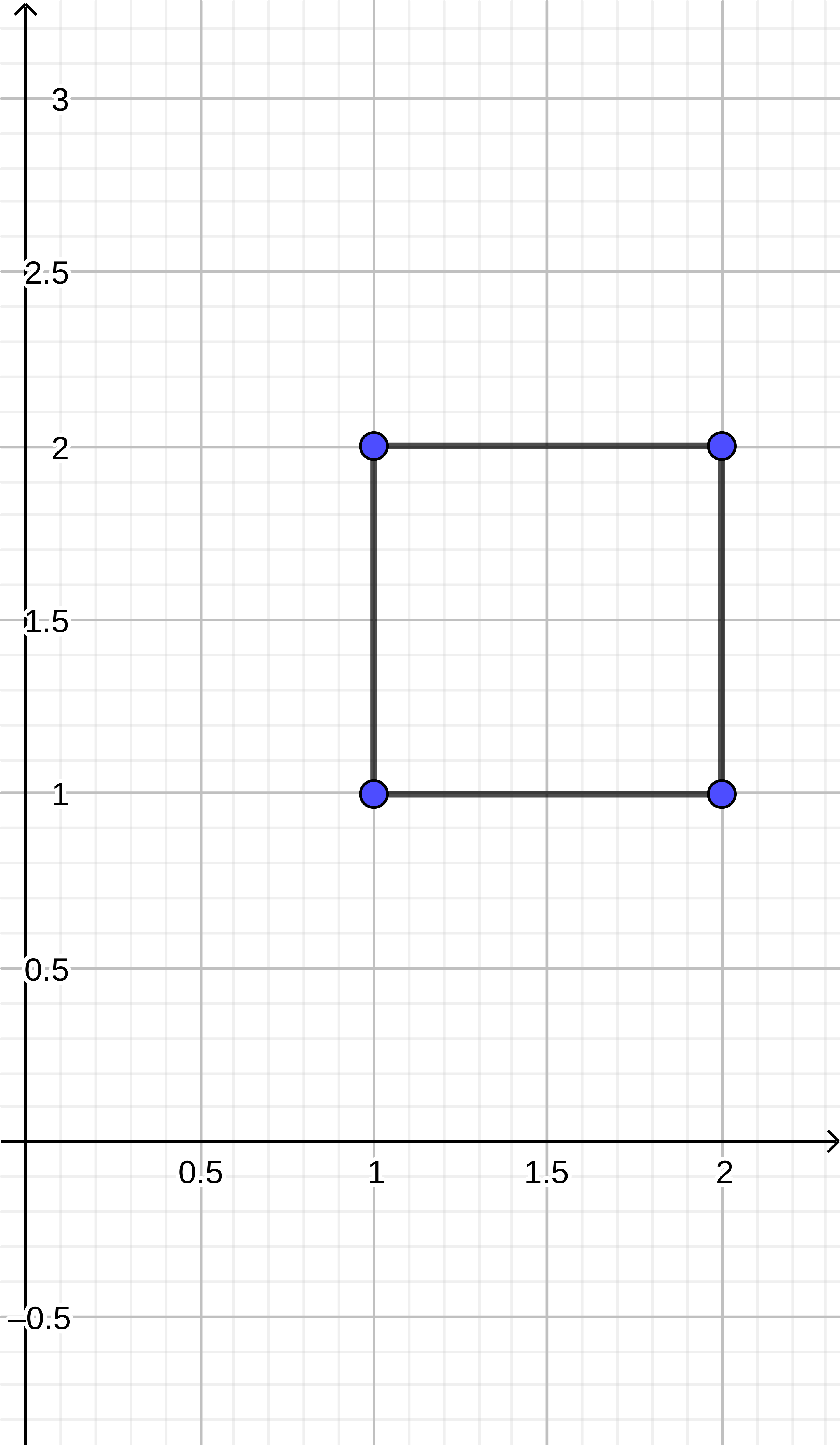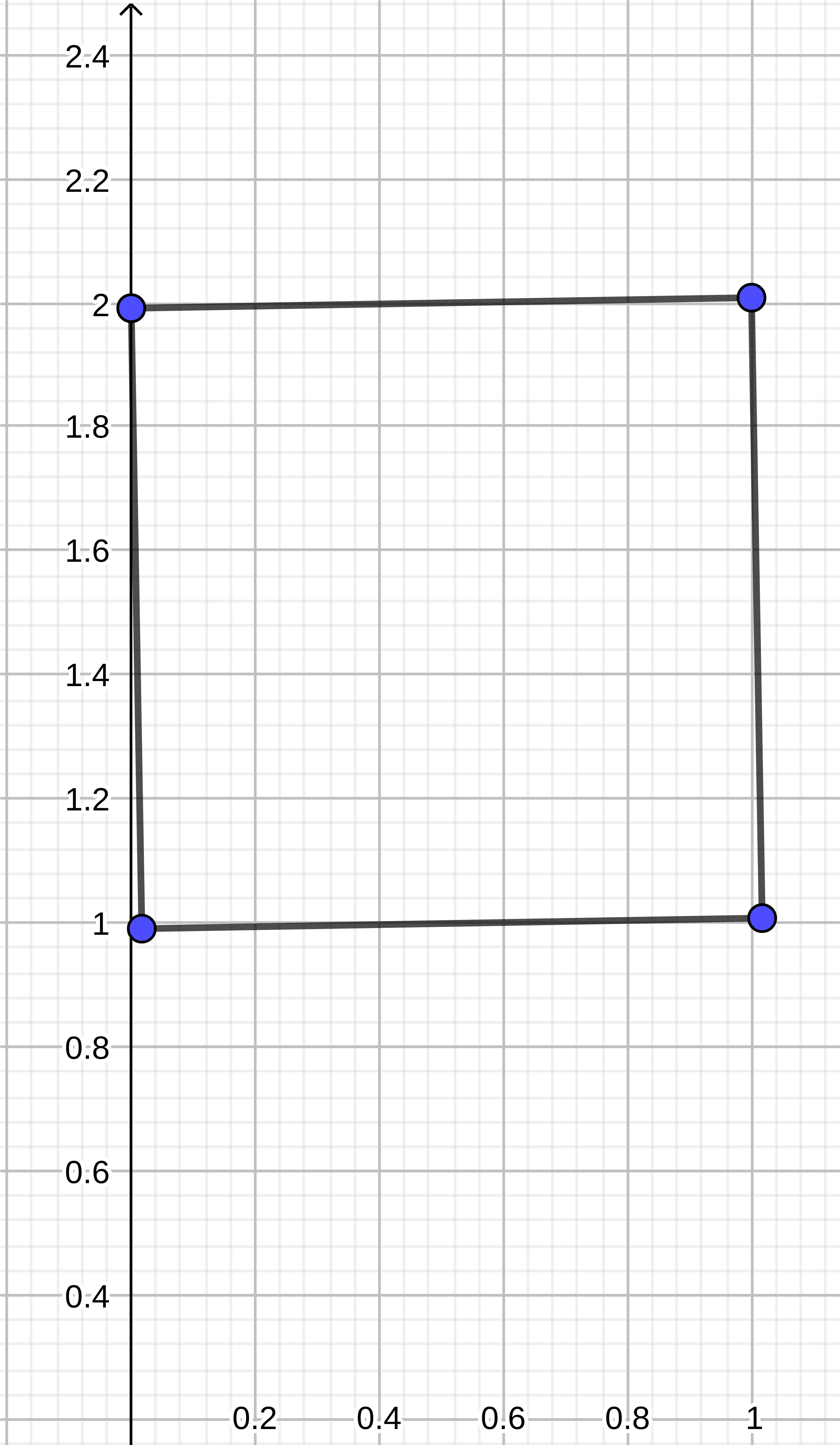Flying square
Tag(s):

## Circles, Geometry, Mathematics, Medium

Problem
Editorial
Analytics

You are given a square. Let's say that the point of intersection of diagonals of the square is a square centre. Square flies to the $Y$-axis in the direction perpendicular to $Y$-axis with the speed $S$ meters per second. Every point of the square rotates by $alpha$ degrees per second clockwise around the centre. Your goal is to find the first moment of time when the square touches the line.

It is guaranteed that tests are numerically stable, i.e. if we change some of the input parameters by a very small value $eps$ $< 10^{-9}$, then the $|newAnswer - answer| < 10^{-6}$.

Input format

Each line $i$ of the 4 subsequent lines (where $1 \le i \le 4$) contains two integers --- $(x_i, y_i)$, $(1 \le x_i, y_i \le 10^{3})$ describing $i$-th vertex of the square in the clockwise or counter-clockwise order.
Next line contains one integer $S$ $(1 \le S \le 10^{3})$ --- speed of the square.
Next line contains one integer $alpha$ $(1 \le alpha < 360)$ --- rotation speed of the square.

Output format

Print one decimal number --- time needed for the square to touch the $Y$-axis with exactly 6 digits after the decimal point

SAMPLE INPUT
2 2
2 1
1 1
1 2
1
1

SAMPLE OUTPUT
0.991423

Explanation

Before rotation:After rotation, at time moment $0.991423$Time Limit: 5.0 sec(s) for each input file.
Memory Limit: 256 MB
Source Limit: 1024 KB
Marking Scheme: Marks are awarded when all the testcases pass.
Allowed Languages: Bash, C, C++, C++14, Clojure, C#, D, Erlang, F#, Go, Groovy, Haskell, Java, Java 8, JavaScript(Rhino), JavaScript(Node.js), Julia, Kotlin, Lisp, Lisp (SBCL), Lua, Objective-C, OCaml, Octave, Pascal, Perl, PHP, Python, Python 3, R(RScript), Racket, Ruby, Rust, Scala, Swift, Swift-4.1, TypeScript, Visual Basic

## CODE EDITOR

Initializing Code Editor...

## This Problem was Asked inChallenge Name

June Easy' 19

OTHER PROBLEMS OF THIS CHALLENGE
• Basic Programming > Input/Output
• Algorithms > Sorting
• Algorithms > Greedy Algorithms
• Algorithms > Graphs
• Algorithms > Graphs